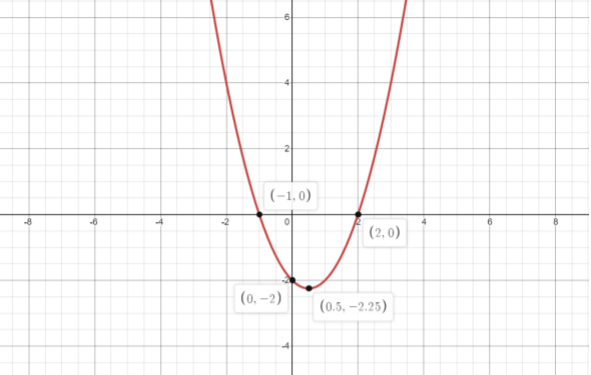Courses
Courses for Kids
Free study material
Offline Centres
MoreLast updated date: 01st Dec 2023
Total views: 282k
Views today: 6.82k

# Factorise the algebraic expression ${z^2} - z - 2$.Verified
282k+ views
Hint: For factoring the given expression we have to find the roots or follow factorization process.
Method 1: FACTORIZATION: In this process we spit the z term into the sum of any two appropriate numbers whose product results in the constant value of the expression. From here we can simplify the rest of the expression.
Method2: GETTING ROOTS: In this method, we find the roots of the expression. We already know that for roots a, b the expression is $(x - a)(x - b)$ and its elaborate form is ${x^2} - (a + b)x + ab$.
Here in this question we take roots be a, b. As from the equation above we know the sum of roots and product of roots . We can get a-b from the formula
${(a - b)^2} = {(a + b)^2} - 4ab$
Now from a + b and a – b we get the values of a and b and substitute them in $(x - a)(x - b)$.

Complete step-by-step solution:
METHOD 1: -z can be written as the sum of -2z and z.
${z^2} - z - 2 \\ = {z^2} - 2z + z - 2 \\ = z(z - 2) + (z - 2) \\ = (z + 1)(z - 2)$
Hence, ${z^2} - z - 2 = (z + 1)(z - 2)$.
METHOD 2 :
Let us consider the roots of expression ${z^2} - z - 2$ be a, b.
By comparison with ${x^2} - (a + b)x + ab$,
$a + b = 1 \\ ab = - 2$
Then,
${(a - b)^2} = {(1)^2} - 4( - 2) \\ \Rightarrow {(a - b)^2} = 1 + 8 \\ \Rightarrow {(a - b)^2} = 9 \\ \Rightarrow a - b = 3 \\ \Rightarrow a = 3 + b$
Substitute the arrived value ‘a’ in a + b , we get
$a + b = 1 \\ \Rightarrow 3 + b + b = 1 \\ \Rightarrow 3 + 2b = 1 \\ \Rightarrow 2b = - 2 \\ \Rightarrow b = - 1$
and
$a = 3 + b \\ \Rightarrow a = 3 + ( - 1) \\ \Rightarrow a = 2$
The expression
${z^2} - z - 2 \\ = (z - a)(z - b) \\ = (z - 2)(z - ( - 1)) \\ = (z - 2)(z + 1)$

Note: The graph of expression ${z^2} - z - 2$ isFrom the graph the roots are ( -1 , 0 ) , ( 2 , 0 ) . From the graph the minimum of the expression ${z^2} - z - 2$ is ( 0.5 , -2.25 ) . Graphical methods are another way to find the roots. The points where the curve intersects the X-axis are roots.